# § 6. Topographical maps (textbook)

§ 6. Topographical maps

1. Remember that you have large scale maps.

2. As called lines, which form a grid on the map of Degree?

How to create a topographical maps. Topographic called zahalnoheohrafichnilarge scale maps (1: 200 000 and more). Distortion at them practically absent, topography given in some detail. Therefore, topographic maps used for detailed study areas and targeting it, precise measurements and calculations, construction design, conduct military exercises, hiking. To use the population issued topokarty all regions of Ukraine in scale 1: 200 000 and 1: 100 000.

The basis topographic maps is the so-called international millionth world map - topographical survey and map of all the earth land, drawn in scale 1: 1000 000. When creating such maps used orthomorphic cylindrical projection, which gives extremely small distortion of shapes and sizes of objects depicted. This surface Earth does not reflect all at once, and some bands (zones), with a width of 6 ° longitude. Each strip design on the side surface imaginary cylinder tangent to the earth's surface along the meridian area. "Turning" the globe and cylinder around the earth's axis R1Р2, Shestyhradusni zone designing sequentially one by one. Then the surface of the cylinder in deploying plane. Designed zone placed near one another, together they will touch only one point - at the equator. But if the glue between all of them themselves, they form a nearly globular shape.

Of course, medium and large-scale maps, if they make for large-size areas will be very cumbersome. For the convenience of their divided into separate sheets, so they called bahatoarkushevymy. A sheet of one million card produced by rozhraflennya globe meridians at 6-degree columns (zones) and parallels 4-degree series. Thus, each sheet map of scale 1:1000 000 looks like a trapezoid the 4 ° in latitude and 6 ° of longitude. The rows and columns are marked accordingly in Latin letters and Arabic numbers. For example, leaf million card that depicts Kyiv, will have a range of M-36.

For to map scale 1: 100 000 million card trapezoid divide meridians and parallels to 144 smaller trapezoids (each of 30 'in longitude and 20 'in latitude). Map sheets each scale (1:50 000, 1:25 000 and 1:10 000) maps are obtained by dividing each of the previous smaller scale 4 trapezoid.

This way, all sheets of topographic maps have borders in the form of trapezoids. Upper (North) and bottom (south) side of the frame are parallel and side (Western and eastern) - meridians. At angles beyond the tops of signed values these parallels and meridians.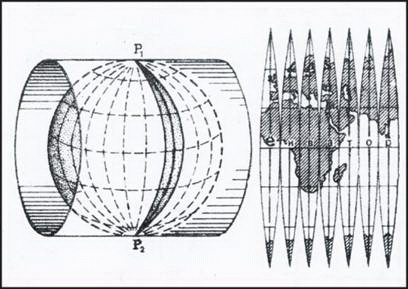Fig. Projection topographic maps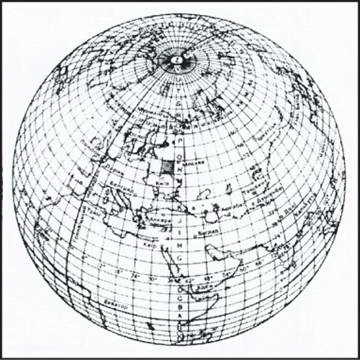Fig. Rozhraflennya globe to create survey and topographic map 1:1000000

Determination geographic coordinates. As is known, parallels and meridians are elements of degree grid, through which the geographic coordinates (longitude and latitude) of any object. For topographic map can be determined from great accuracy. For this frame topokarty divided on the interval equal to 1 '(with turns one and two fatty thin parallel lines). At each interval minutnomu points indicate point value equal to 10 ". So, to find geographical coordinates of any point, we should hold through to the two parties beyond maps lines that correspond to the parallels and meridians, and read the value on the frame latitude and longitude to within seconds.

Determination rectangular coordinate. On topographic maps drawn as rectangular (Km) grid, which allows you to set the rectangular coordinates any point on the map. Cartesian coordinates - A coordinate system in which axis X adopted 6-axis meridian degree zone, and the axis Y - Equator. These two lines (axial meridian and the equator) in the design area on the surface cylinder are mutually perpendicular straight lines, other meridians and parallels are curves. The point of intersection of the axial meridian and the equator is the beginning of rectangular coordinates of each zone.

Rectangular coordinates show the distance in kilometers to this point from the equator (coordinate XWhich can range from 0 to more than 10 000 km at the poles) and from initial line (coordinate YWhich can range from 0 to 333 km equator where its intersection with the extreme western and eastern meridians zone).

On topographic maps marked with lines that carried through every 1 or 2 miles and parallel axes X and Y. They form a kilometer grid that card system covers an area equal in square. Near the framework maps signed value line kilometer grid. Double figures presented large numbers at the horizontal and vertical lines are used to indicate the square, which is the required point. In this first recorded the lower number of horizontal lines of the square, then the number left vertical line. If you need to better define the position of the point inside the square, then determine its rectangular coordinates to the nearest meter.

For that from this point perpendicular to spend the southern and western sides square and using the scale measure the distance to them. These values add numbers to the appropriate kilometer lines. Thus, point B will coordinates: X = 80462, Y = 09655.

Using kilometer grid topographic map, you can also reverse the problem: put on the map point on known coordinates. For example, if point has rectangular coordinates X = 81 450, Y = 08 780, it need to put in the box 8108. point will be located at a distance of 450 m north of lower horizontal line Square and within 780 m east of left vertical line. Having in this square are two lines at the appropriate distances from such parties square, we find the point in the place searched intersection of these lines.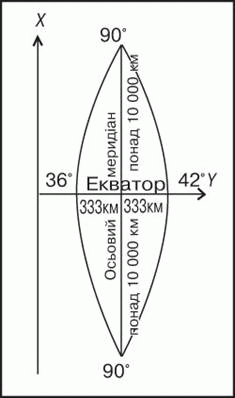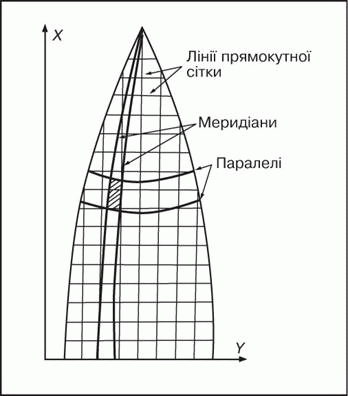Fig. Grid Zone coordinates

Remember

Topographic called zahalnoheohrafichni big map scale (1: 200 000 and more), where topography is transferred detail.

By topographic map can be determined with high accuracy geographical coordinates (Longitude and latitude) of any object.

For topographical cards (per kilometer rectangular grid) can be set square coordinates anywhere or cause any point on the map with its rectangular coordinates.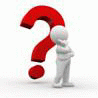Questions and Tasks

1. What is a topographic map? How does it differ from the physical maps Ukraine in your atlas?

2. How to create topographic maps?

3. Find out where rows and columns (zones), according to an international scheme rozhraflennya globe, is the territory of Ukraine.

4. How to determine the rectangular coordinates for the topographic map?